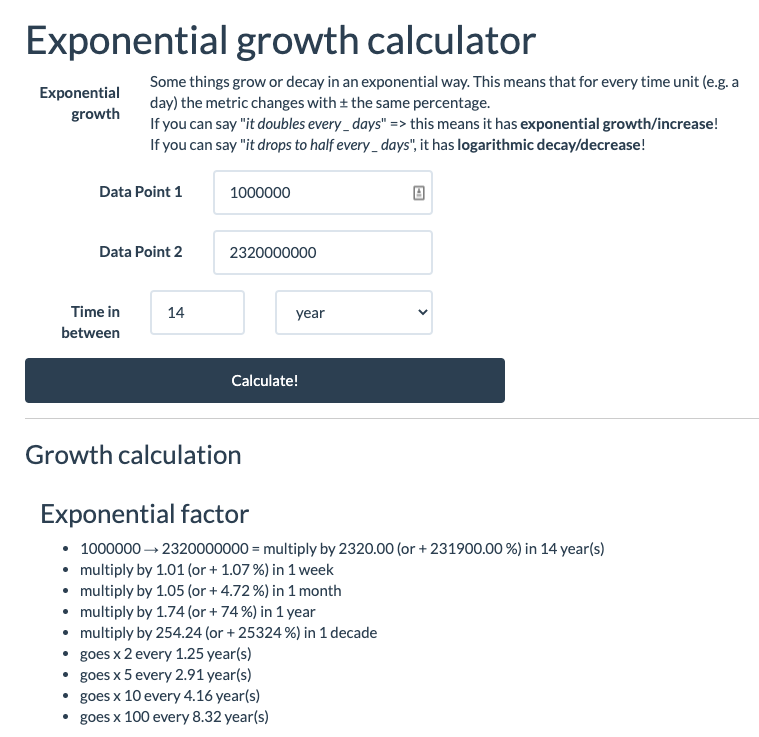# Calculate exponential growth trends

There has been a lot of talk about exponential growth the last few weeks, regarding the spread of COVID-19. It can be a tough concept to get your head around. If you hear “It doubles every week”, ok so that means what for next month? Times 8? Times 16? I wanted to make an easy calculate exponential (going up) and logarithmic (going down) phenomena.

Introducing the Exponential Growth Calculator! Just add two data points and let the tool extrapolate.

Some examples: